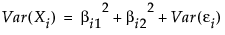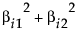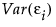Consider a situation where you have ten observed variables, X1, X2, …, X10. Suppose that you want to model these ten variables in terms of two latent factors, F1 and F2. For convenience, it is assumed that the factors are uncorrelated and that each has mean zero and variance one. The model that you want to derive is of the form:
It follows that. The portion of the variance of Xi that is attributable to the factors, the common variance or communality, is. The remaining variance,, is the specific variance, and is considered to be unique to Xi.

Help created on 9/19/2017